# Forecasting time series with ARIMAPosted by

In this post, I’ll attempt to show how to forecast time series data using ARIMA (autoregressive integrated moving average). As usual, I try to practice with “real-world”, which can be obtained easily by downloading open data from government websites.

I chose the unemployment rate in the European Union’s 27 member countries. The data were obtained from the OECD data portal (http://dataportal.oecd.org/).

First of all, I’m going to try to clean up the data, in this case, it’s rather easy, and then try to modify it so that the data can be used in an ARIMA model. Finally, I will try to figure out how well the model fits our data.

Great explanations on the ARIMA model can be found on many internet sites, but I will present a brief summary here.

AR Term: AR is Auto Regressive. This is the number of previous observations that are correlated with the current one. (partial autocorrelation). This is the p parameter in the model (number of lags with significant correlation with the current observation).

I Term: I am Integrated. ARIMA needs the time series to be stationary in order to work properly, that is the data shouldn’t have trend or seasonality. If the data has a trend we must transform it in order to model this trend. Usually what we do is differencing, that is, modeling the difference between an observation and the previous one (or the nth previous ones) rather than the actual values. This is the parameter d in the model, which means the order of differencing.

MA Term: MA is Moving Average. It accounts for the error in the previous lags, that is, we take into account the errors (residuals) in the previous observations (difference between the expected and the actual data) to predict the future values. This is the parameter q in the model, which is the order of the moving average (the number of previous observations to take into account in the moving average).

European_Countries_Unemployment_Data

Let's import the necessary libraries

In :
import pandas as pd
import glob
import numpy as np
import seaborn as sn
import matplotlib.pyplot as plt
from statsmodels.graphics.tsaplots import plot_pacf, plot_acf
from statsmodels.tsa.statespace.sarimax import SARIMAX
from statsmodels.tsa.stattools import pacf


In :
from google.colab import drive
drive.mount('/content/drive')

Drive already mounted at /content/drive; to attempt to forcibly remount, call drive.mount("/content/drive", force_remount=True).


Let's import the data, before doing it I've had to remove the double quotes from the csv file.

In :
data = pd.read_csv('/content/drive/MyDrive/DP_LIVE_10082021082010744.csv', parse_dates=['TIME'], index_col='TIME', header=0,sep=",")

Out:
LOCATION INDICATOR SUBJECT MEASURE FREQUENCY Value Flag Codes
TIME
2000-01-01 EU27_2020 HUR TOT PC_LF M 9.2 NaN
2000-02-01 EU27_2020 HUR TOT PC_LF M 9.2 NaN
2000-03-01 EU27_2020 HUR TOT PC_LF M 9.2 NaN
2000-04-01 EU27_2020 HUR TOT PC_LF M 9.1 NaN
2000-05-01 EU27_2020 HUR TOT PC_LF M 9.1 NaN

Let's remove the unnecessary columns, we are only interested in TIME and Value

In :
data.drop(['LOCATION','INDICATOR','SUBJECT','MEASURE','FREQUENCY','Flag Codes'],axis=1,inplace=True)


Let's plot the data

In :
data.plot(figsize=(15,5))

Out: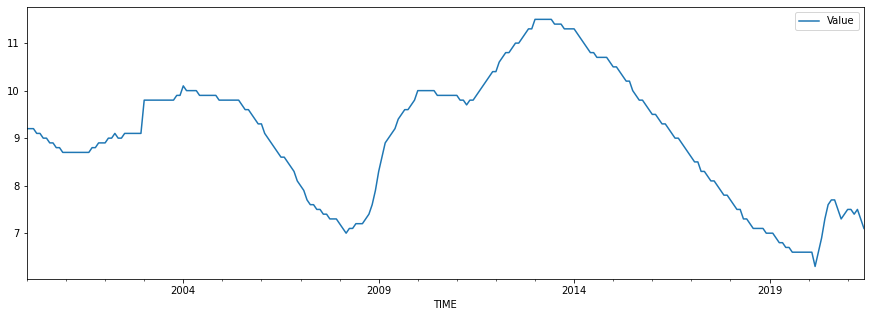The first step is to check for stationarity. ARIMA models require stationary data, which means that the values in the time series do not depend on the time at which the data is observed. The variance in a stationary time series is constant. The Dickey–Fuller test will be used. The null hypothesis here is that the data contains a unit root, implying that the time series is not stationary. Because the p-value in the results is greater than 0.05, we cannot reject the null hypothesis (there is a unit root).

In :
from statsmodels.tsa.stattools import adfuller
X = data.Value
print('p-value: %f' % result)
print('Critical Values:')
for key, value in result.items():
print('\t%s: %.3f' % (key, value))

ADF Statistic: -1.925368
p-value: 0.320223
Critical Values:
1%: -3.457
5%: -2.873
10%: -2.573


Let's see what happens if we difference the data to make it stationary.

In :
data_diff=data.diff().dropna()


## The trend in the data has been removed, as shown in the plot.¶

In :
data_diff.plot(figsize=(15,5))

Out: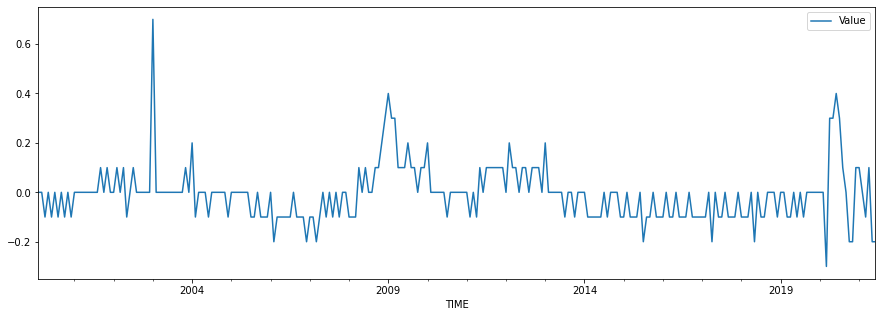On the differenced data, run the Dickey-Fuller test. As we can see, the p-value now allows us to reject the null hypothesis, allowing us to conclude that the data is stationary.

In :
from statsmodels.tsa.stattools import adfuller
dataX = data_diff.Value
print('p-value: %f' % fuller)
print('Critical Values:')
for key, value in fuller.items():
print('\t%s: %.3f' % (key, value))

ADF Statistic: -2.987032
p-value: 0.036123
Critical Values:
1%: -3.457
5%: -2.873
10%: -2.573


Let us now examine the autocorrelation and partial autocorrelation plots. The autocorrelation plot (which assists us in determining the MA term) shows a gradually decreasing trend, indicating that no MA term is required, whereas the partial autocorrelation plot (which assists us in determining the AR term) drops abruptly after two lags (we do not count the first lag). This implies that we should use an AR(2) model.

In :
plot_acf(np.array(data_diff))
plt.show()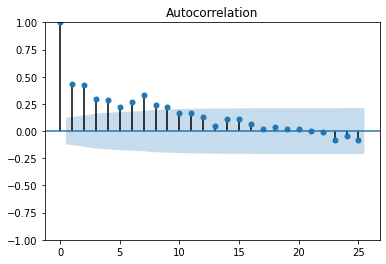In :
plot_pacf(np.array(data_diff))
plt.show()

/usr/local/lib/python3.7/dist-packages/statsmodels/graphics/tsaplots.py:353: FutureWarning: The default method 'yw' can produce PACF values outside of the [-1,1] interval. After 0.13, the default will change tounadjusted Yule-Walker ('ywm'). You can use this method now by setting method='ywm'.
FutureWarning,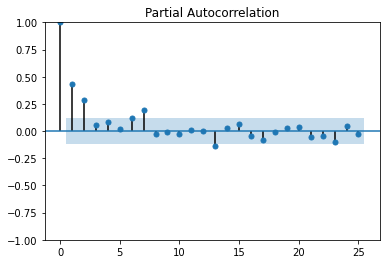In :
#In google colab we should install pmdarima in order to use it.
!pip install pmdarima

Requirement already satisfied: pmdarima in /usr/local/lib/python3.7/dist-packages (1.8.3)
Requirement already satisfied: joblib>=0.11 in /usr/local/lib/python3.7/dist-packages (from pmdarima) (1.0.1)
Requirement already satisfied: numpy>=1.19.3 in /usr/local/lib/python3.7/dist-packages (from pmdarima) (1.19.5)
Requirement already satisfied: Cython!=0.29.18,>=0.29 in /usr/local/lib/python3.7/dist-packages (from pmdarima) (0.29.24)
Requirement already satisfied: scikit-learn>=0.22 in /usr/local/lib/python3.7/dist-packages (from pmdarima) (0.22.2.post1)
Requirement already satisfied: statsmodels!=0.12.0,>=0.11 in /usr/local/lib/python3.7/dist-packages (from pmdarima) (0.13.0)
Requirement already satisfied: scipy>=1.3.2 in /usr/local/lib/python3.7/dist-packages (from pmdarima) (1.4.1)
Requirement already satisfied: urllib3 in /usr/local/lib/python3.7/dist-packages (from pmdarima) (1.24.3)
Requirement already satisfied: setuptools!=50.0.0,>=38.6.0 in /usr/local/lib/python3.7/dist-packages (from pmdarima) (57.4.0)
Requirement already satisfied: pandas>=0.19 in /usr/local/lib/python3.7/dist-packages (from pmdarima) (1.1.5)
Requirement already satisfied: pytz>=2017.2 in /usr/local/lib/python3.7/dist-packages (from pandas>=0.19->pmdarima) (2018.9)
Requirement already satisfied: python-dateutil>=2.7.3 in /usr/local/lib/python3.7/dist-packages (from pandas>=0.19->pmdarima) (2.8.2)
Requirement already satisfied: six>=1.5 in /usr/local/lib/python3.7/dist-packages (from python-dateutil>=2.7.3->pandas>=0.19->pmdarima) (1.15.0)
Requirement already satisfied: patsy>=0.5.2 in /usr/local/lib/python3.7/dist-packages (from statsmodels!=0.12.0,>=0.11->pmdarima) (0.5.2)


Let's try it. First we should split the data into train and test sets.

In :
TEST_SIZE = 36
train, test = data.iloc[:-TEST_SIZE], data.iloc[-TEST_SIZE:]
x_train, x_test = np.array(range(train.shape)), np.array(range(train.shape, data.shape))
train.shape, x_train.shape, test.shape, x_test.shape

Out:
((222, 1), (222,), (36, 1), (36,))
In :
fig, ax = plt.subplots(1, 1, figsize=(15, 5))
ax.plot(x_train, train)
ax.plot(x_test, test)

Out:
[]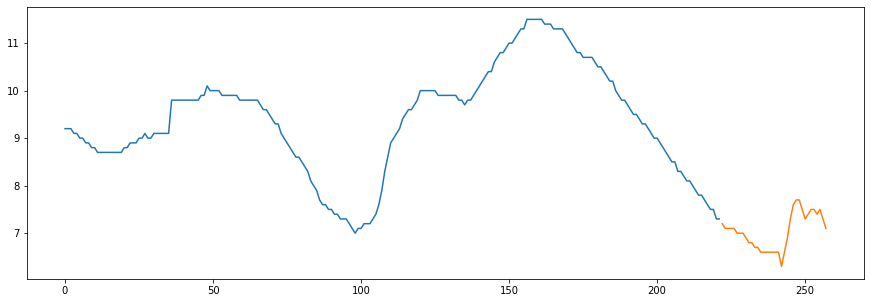In :
from pmdarima.arima import ARIMA
from pmdarima.arima import ndiffs
from pmdarima.arima import nsdiffs


Let's check for the need for differencing and seasonally differencing the data using the ndiffs and nsdiffs functions.

First, the ndiffs function attempts to predict the order of differencing required for the data to be stationary; as we saw with the Dickey-Fuller tests, the data requires one order of differencing to be stationary.

In :
ndiffs(data)

Out:
1

The nsdiffs function displays the D parameter, which determines whether the data requires seasonal differencing. We chose 12 as the m parameter because we have monthly data (The seasonal period to check). The result is 0, as expected, because the data is already seasonally adjusted, so we don't need to do anything about seasonality.

In :
nsdiffs(data,12)

Out:
0

Let's fit now the ARIMA model. The p parameter is 2 (AR(2)), the d parameter is 1 (1 order of differencing), the q parameter in this case is 0 (no MA model).

In :
model = ARIMA(order=(2,1,0))
results = model.fit(train)

In :
model.summary()

Out:
Dep. Variable: No. Observations: y 222 SARIMAX(2, 1, 0) 228.519 Tue, 05 Oct 2021 -449.037 08:25:01 -435.445 0 -443.549 - 222 opg
coef std err z P>|z| [0.025 0.975] -0.0032 0.007 -0.441 0.659 -0.018 0.011 0.2641 0.044 6.040 0.000 0.178 0.350 0.3933 0.036 10.899 0.000 0.323 0.464 0.0074 0.000 28.176 0.000 0.007 0.008
 Ljung-Box (L1) (Q): Jarque-Bera (JB): 0.74 3646.6 0.39 0 0.4 2.46 0 22.28

Warnings:
 Covariance matrix calculated using the outer product of gradients (complex-step).

According to the model summary:

• Because the p-value of the Ljung-Box test is greater than 0.05, we cannot reject the null hypothesis that the residuals are independent.

• Because the p-value of the heteroskedasticity test is less than 0.05, we can reject the null hypothesis that the error variances are equal. Because this is an assumption for a correct ARIMA model, we cannot completely rely on these results.

Let's make the prediction of the three years of test data and compare it.

In :
# Forecast
prediction, confint = model.predict(n_periods=TEST_SIZE, return_conf_int=True)
prediction

Out:
array([7.21812198, 7.19328326, 7.15130377, 7.12723166, 7.10114674,
7.0815733 , 7.06292766, 7.04708799, 7.03235417, 7.01901595,
7.0064812 , 6.99470751, 6.98345078, 6.97262989, 6.96212741,
6.95188043, 6.94182615, 6.93192324, 6.92213608, 6.91243903,
6.90281131, 6.89323732, 6.88370479, 6.87420434, 6.86472867,
6.85527217, 6.84583046, 6.8364002 , 6.82697879, 6.81756421,
6.80815492, 6.79874971, 6.78934766, 6.77994805, 6.77055032,
6.76115405])

The graph below compares the predictions and the test data. The grey zone represents the prediction's confidence interval, and as we can see, the observed data fits well within it.

In :
cf= pd.DataFrame(confint)
prediction_series = pd.Series(prediction,index=test.index)
fig, ax = plt.subplots(1, 1, figsize=(15, 5))
ax.plot(data.Value)
ax.plot(prediction_series)
ax.fill_between(prediction_series.index,
cf,
cf,color='grey',alpha=.3)

Out: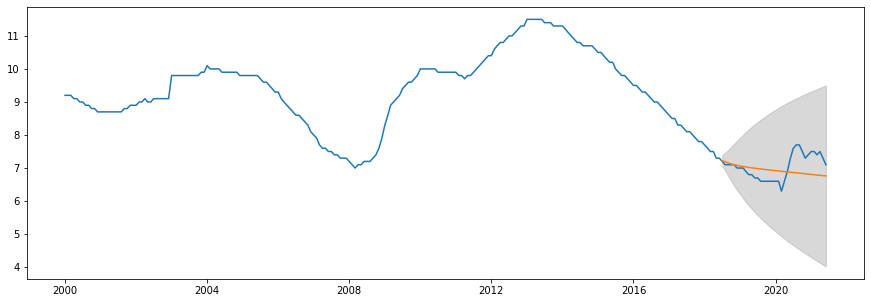Finally, consider SMAPE (Symmetric Mean of Absolute Percentage Errors), which is similar to MAPE but avoids the issue of penalizing negative errors (actual < forcasted).

In :
def calcsmape(actual, forecast):
return 1/len(actual) * np.sum(2 * np.abs(forecast-actual) / (np.abs(actual) + np.abs(forecast)))

In :
smape=calcsmape(test.Value,prediction)
smape

Out:
0.05145800665592428

Let's try with 1 year of data instead of three.

In :
TEST_SIZE = 12
train, test = data.iloc[:-TEST_SIZE], data.iloc[-TEST_SIZE:]
x_train, x_test = np.array(range(train.shape)), np.array(range(train.shape, data.shape))
train.shape, x_train.shape, test.shape, x_test.shape
fig, ax = plt.subplots(1, 1, figsize=(15, 5))
ax.plot(x_train, train)
ax.plot(x_test, test)

Out:
[]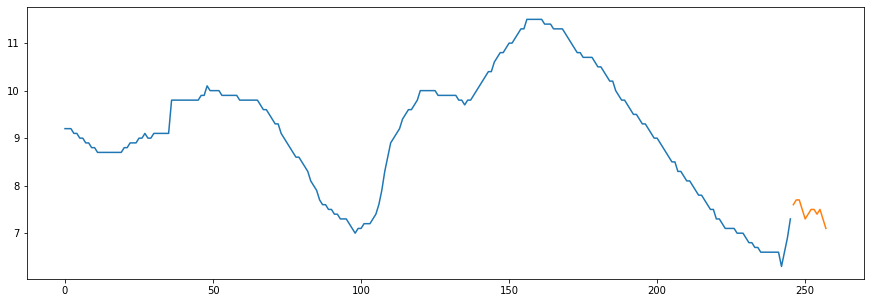In :
model1y = ARIMA(order=(2,1,0))
results1y = model1y.fit(train)
# Forecast
prediction, confint = model1y.predict(n_periods=TEST_SIZE, return_conf_int=True)
prediction

Out:
array([7.52485398, 7.73496936, 7.87703065, 7.99385755, 8.07854597,
8.14466595, 8.19366651, 8.23091006, 8.25848968, 8.27896832,
8.29385614, 8.30452695])
In :
cf= pd.DataFrame(confint)
prediction_series = pd.Series(prediction,index=test.index)
fig, ax = plt.subplots(1, 1, figsize=(15, 5))
ax.plot(data.Value)
ax.plot(prediction_series)
ax.fill_between(prediction_series.index,
cf,
cf,color='grey',alpha=.3)

Out: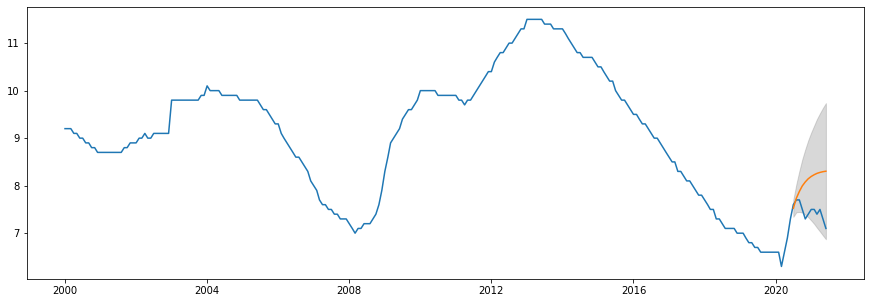In :
model1y.summary()

Out:
Dep. Variable: No. Observations: y 246 SARIMAX(2, 1, 0) 236.568 Tue, 05 Oct 2021 -465.135 08:25:02 -451.130 0 -459.496 - 246 opg
coef std err z P>|z| [0.025 0.975] -0.0011 0.007 -0.158 0.874 -0.015 0.013 0.2920 0.035 8.424 0.000 0.224 0.360 0.3640 0.036 10.012 0.000 0.293 0.435 0.0085 0.000 26.482 0.000 0.008 0.009
 Ljung-Box (L1) (Q): Jarque-Bera (JB): 0.29 2348.02 0.59 0 0.81 2.14 0.35 17.55

Warnings:
 Covariance matrix calculated using the outer product of gradients (complex-step).

The model summary states:

• We continue to hold true to the assumption that the residuals are not autocorrelated (Ljung-Box test)

• We can also reject the null hypothesis of heteroskedasticity in the residuals this time (Prob(H) 0.05), making these results more reliable than the 3-year prediction results.

In :
smape=calcsmape(test.Value,prediction)
smape

Out:
0.08096459003790507

Conclusion

We learned how to obtain data from official sources so that we could test our data science skills with real-world data. The EU-27 unemployment figures were obtained from the OECD’s official website. We practiced with time series data and an ARIMA model this time. We first checked for stationarity in the data, then used differencing to make it stationary, and finally used autocorrelation and partial autocorrelation plots to estimate the ARIMA model parameters. Finally, using these parameters, we ran the model and validated it using the SMAPE metric and the autocorrelation plot of squared residuals (Symmetric Mean Absolute Error).

We also interpreted the model results summary to see how much we can trust these results. The conclusion is that a one-year prediction yields a better model than a three-year prediction, given that the three-year prediction still has unaccounted for variance in the residuals.